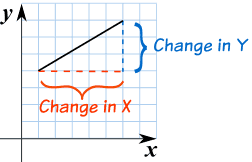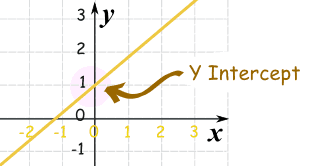# Unit Rate

Standard

Unit rates are about the amount for 1 unit.

We use Ratio Tables to help solve for rates.
Our Ratio table looks like a tic-tac-toe table.We MUST label our table to know where to put our information

Ex. Bob drives 100 miles in 5 hours. How many miles does he travel every hour?Now we fill in the information that is given.

Bob drives 100 miles in 5 hours. How many miles does he travel every hour?Once we have entered what was given we need to see what the question is asking us.

Ex. Bob drives 100 miles in 5 hours. How many miles does he travel every hour?

Ask yourself, is the problem asking how many hours it takes for 1 mile or how many miles for 1 hour???????

If you said how many miles for 1 hour, you are correct!

So now we enter that into our Ratio Table.The x is the unknown. This is what we are solving for.To solve for x, we multiply 100 and 1. Then divide by the number left over which is 5.

We end up with x = 20.

So Bob drives 20 miles per hour.

Click here to watch a video with a different way to find unit rate.

Test yourself on unit rates here!

# Slope Intercept Form

Standard

### y = mx + b

m = Slope (how steep the line is)

b = the Y Intercept (where the line crosses the Y axis)\### How do you find “m” and “b“?

• b is easy: just see where the line crosses the Y axis.
• m (the Slope) needs some calculation. Remember we like to write slope like a fraction.

## Example 1)The fastest and easiest thing to find first when looking at a graph is the y-intercept (b).

Here we see that the line crosses the y-axis at positive 1.

So, b = 1 .

Now to find the slope (m) we will use rise over run:

• Rise is how far up
• Run is how far along

In this example the rise is 2 and the run is 1.

So, m = 2/1 .

Now that I know m = 2/1 and b = 1 I can plug them into the equation for slope intercept form y = mx + b.

y = (2/1) x + 1

^^^^^ This is the equation of the line.

# Slope

Standard
Slope is about how steep a line is.
When finding the slope from a line on a graph we use the method of rise over run .
• Rise is how far up
• Run is how far alongYou can also think of it as the change in y over the change in x.EXAMPLE #1:The slope here is 4/6 which can simplify to 2/3.
EXAMPLE #2:In this example the slope is 3/5.

# Practice Problems

TEST YOUR SELF ON LINES HERE!!!!

# Y – intercept

Standard

## Y Intercept

Where a straight line crosses the Y axis of a graph.

### Example:In the above diagram the line crosses the Y axis at 1.

So the Y intercept is equal to 1.

## Practice Problems

TEST YOUR SELF ON LINES HERE!!!!

# UO Video for Unit 4

Standard

The audio is a bit quiet, so make sure you have the speakers set up to high.

# Interest

Standard

### Introduction to interest:

http://www.mathsisfun.com/money/interest.html

SIMPLE INTEREST

I = Prt

• I = interest  [\$]
• P = amount borrowed (called “Principal”)  [\$]
• r = interest rate   [%]
• t = time    [years]

COMPOUND INTEREST

A = P(1+r)^t

• A = actual amount owed (total debt)   [\$]
• P = amount borrowed (called “Principal”)    [\$]
• r = interest rate     [%]
• t = time    [years]

Information on compound interest:

http://www.mathsisfun.com/definitions/compound-interest.html

SIMPLE INTEREST PRACTICE PROBLEMS:

http://www.mathopolis.com/questions/q.php?id=1702&site=1&ref=/money/interest.html&qs=1702_1703_1704_1705_3749_3750_3751_1706_1707_1708

COMPOUND INTEREST PRACTICE PROBLEMS:

http://www.mathopolis.com/questions/q.php?id=2294&site=1&ref=/money/compound-interest.html&qs=2294_2295_2296_2297_2298_2299_2300_2301_2302_2303